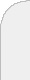inicioir al DIIbuscarshape-constrained optimization Various statistical estimation and learning theory problems, as well as problems in engineering, economics, finance, and geometry can be formulated as convex optimization problems with side constraints on the shape of some underlying function. Such constraints include nonnegativity, monotonicity, and convexity. After presenting a number  of applications, we shall discuss approaches for the efficient solution of these models.The shape constraints can be conveniently formulated in the conic programming framework, and if the shape-constrained function is assumed to belong to a "nice" set, such as polynomials, splines, or trigonometric polynomials, the models simplify further, to semidefinite or second-order conic programming models. In other cases, good approximations can be obtained by similar conic programming models. I will present a broad overview of the basics of conic programming, prior knowledge is not required.